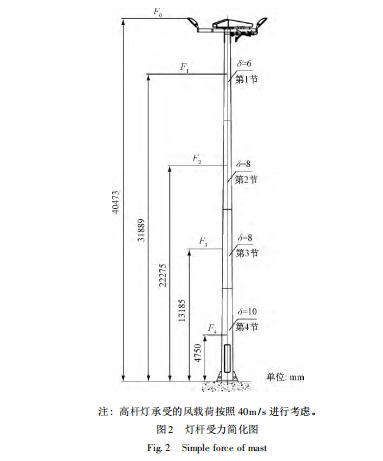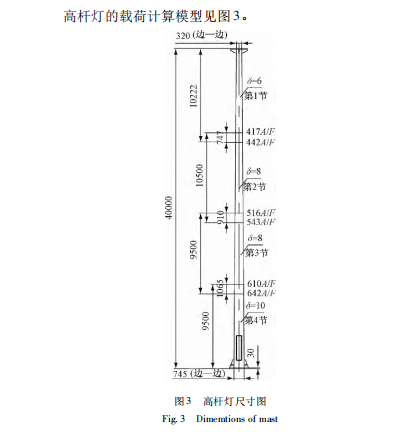0514-84242547# 40米高杆灯风载荷的设计计算与校核

( 1) 永久载荷: 结构自重、固定的设备重、物料重、土重、土压力、线的拉力等。

( 2) 可变载荷: 风载荷、裹冰载荷、常见地震作用、雪载荷、安装检修载荷、塔楼楼面或平台的活载荷、温度变化、地基沉陷等。

( 3) 偶然载荷: 导线断裂、索线断线，撞击、爆炸、罕遇地震作用等。雪荷载为施加在建筑屋面或其他结构外露面上的积雪重量。雪荷载值 S 由地面积雪重量即基本雪压 So 乘以屋面积雪分布系数 μr 确定:

S = μrSo［3］

裹冰荷载对于如输电塔架、线路等结构往往是一种重要荷载。由于裹冰增大了杆件、缆索的截面，或封闭了某些格构的空隙，不但使结构或构件的重量增大，而且由于结构挡风面积增大，显著地加大了风荷载，使结构受力更为不利。地震载荷对高耸结构的破坏是非常严重的，而且该破坏往往还隐藏着潜在危险。

( 1) 静力理论。该理论不考虑建筑物的动力特性，假设结构物为绝对刚性，地震时建筑物运动与地面运动绝对一致，建筑物的最大加速度等于地面运动的最大加速度，建筑物所受的最大载荷等于其质量与地面最大加速度的乘积。该理论只适用于低矮的，刚性较大的建筑物。

( 2) 反应谱理论。它既考虑了地震时地面的动力特性，也考虑了结构自身的动力特性，是当前工程设计应用最为广泛的地震设计方法之一。反应谱理论是以单质点体系在实际地震作用下的反应为基础来分析结构反应的方法。抗震设计中通常只需地震作用下的最大载荷值，其值为:

F = mamax = w ( g ) amax 3式中，w 为质点质量，称为水平地震影响因素。

( 3) 直接动力分析理论。反应谱在分析大跨度的柔性结构时，由于非线性因素的影响，反应谱方法的计算误差较大。直接动力分析理论可以克服反应谱理论的缺点。可直接获得地震过程中结构节点各时刻位移，速度，加速度，从而计算各时刻竖向

温度作用应考虑气温变化、太阳辐射及使用热源等因素。作用在结构或构件上的温度作用应采用其温度的变化来表示。计算结构或构件的温度作用效应时，应采用材料的线性热膨胀系数。对于大型或超大型，由不同材料部件组成的结构件，应同时考虑不同部件材料之间的温度作用和整个结构件温度场的变化。

1 设计要求2 高杆灯风载荷计算高杆灯的风载荷在各个不同高度处均不同，因此，为了简便起见，我们可以将高杆灯的每一节视

F3、F4 ) ，就可以近似得到整个高杆灯的风载荷。126 照明工程学报 2014 年 10 月Fig. 3 Dimemtions of mast作用在高杆灯上单位面积上的风载荷应按照下式计算:

ωh = βzμsμzμrωo［2］ ( 1)

2. 1 基本风压 ωo

( 2)式中，V0 为设计风速 ( 单位: m / s) 。将已知风速 40 米/秒代入上式，计算得出基本风压为 ωo = 1 kN /m2。

2. 2 不同高度处的风压高度变化系数见表 1

Table 1 Height variation factor of wind pressure μz离地面或海面高度/m地面粗糙度类别

A B C

5 1. 17 0. 80 0. 54

10 1. 38 1. 00 0. 71

15 1. 52 1. 14 0. 84

20 1. 63 2. 25 0. 94

30 1. 80 1. 42 1. 11

40 1. 92 1. 56 1. 24

50 2. 03 1. 67 1. 36

60 2. 12 1. 77 1. 46

2. 3 风振系数

ξ、ε1 ε2 的选取参见表 2 ～ 表 4。0. 01 1. 47 1. 11

0. 05 1. 73 1. 18

0. 10 1. 88 1. 23

0. 20 2. 04 1. 28

0. 40 2. 24 1. 34

0. 60 2. 36 1. 38

0. 80 2. 46 1. 42

1. 00 2. 53 1. 44

2. 00 2. 80 1. 54

4. 00 3. 09 1. 65

6. 00 3. 28 1. 72

8. 00 3. 42 1. 77

10. 00 3. 54 1. 82

20. 00 3. 91 1. 96

30. 00 4. 14 2. 06

Table 3 Influence coefficient of wind pressure pulsationand wind pressure height variation ε1

A B C

10 0. 57 0. 72 0. 93

20 0. 51 0. 63 0. 79

40 0. 45 0. 55 0. 69

60 0. 42 0. 50 0. 59

80 0. 39 0. 45 0. 54

100 0. 37 0. 43 0. 50

150 0. 33 0. 37 0. 43

200 0. 30 0. 34 0. 38

250 0. 27 0. 31 0. 34

300 0. 25 0. 28 0. 29

Table 4 Influence factor for mode，structure and shape ε2

1. 0 0. 5 0. 3 0. 2 0. 1

1. 0 1. 00 0. 88 0. 76 0. 66 0. 56

0. 9 0. 89 0. 83 0. 73( 0. 79) 0. 65( 0. 76) 0. 57( 0. 84)

0. 8 0. 78 0. 76 0. 67( 0. 77) 0. 61( 0. 78) 0. 57( 0. 96)

0. 7 0. 66 0. 66 0. 60( 0. 70) 0. 55( 0. 73) 0. 54( 0. 94)

0. 6 0. 54 0. 56 0. 51( 0. 60) 0. 48( 0. 64) 0. 49( 0. 84)

0. 5 0. 42 0. 44 0. 41( 0. 48) 0. 40( 0. 58) 0. 42( 0. 69)

0. 4 0. 31 0. 32 0. 31( 0. 35) 0. 30( 0. 38) 0. 34( 0. 52)

0. 3 0. 20 0. 22 0. 22 0. 21( 0. 25) 0. 27( 0. 38)

0. 2 0. 11 0. 11 0. 12 0. 13 0. 15( 0. 19)

0. 1 0. 04 0. 04 0. 04 0. 05 0. 06

βz0 = 1 + ξε1ε2 = 1 + 2. 53 × 0. 45 × 0. 88 = 2

βz1 = 1 + ξε1ε2 = 1 + 2. 53 × 0. 45 × 0. 76 = 1. 89

βz2 = 1 + ξε1ε2 = 1 + 2. 53 × 0. 45 × 0. 56 = 1. 64

βz3 = 1 + ξε1ε2 = 1 + 2. 53 × 0. 45 × 0. 32 = 1. 36

βz4 = 1 + ξε1ε2 = 1 + 2. 53 × 0. 45 × 0. 04 = 1. 05

2. 4 各质量单元的单位面积风载荷

ωh0 = βz0μsμz0μrωo = 2 × 0. 7 × 1. 92 × 1. 2 × 1

= 3. 23 ( kN /m2)

ωh1 = βz1μsμz1μrωo = 1. 89 × 0. 7 × 1. 80 × 1. 2 × 1

= 2. 86 ( kN /m2)

ωh2 = βz2μsμz2μrωo = 1. 64 × 0. 7 × 1. 63 × 1. 2 × 1

= 2. 25 ( kN /m2)

ωh3 = βz3μsμz3μrωo = 1. 36 × 0. 7 × 1. 52 × 1. 2 × 1

= 1. 74 ( kN /m2)

ωh4 = βz4μsμz4μrωo = 1. 05 × 0. 7 × 1. 17 × 1. 2 × 1

= 1. 03 ( kN /m2)

2. 5 各质量单元的风载荷作用力 F

F = ωhi × Si

［1］ ( 4)

F0 = ωh0 × S0 = 3. 23 × 4. 17 × 0. 473 × 0. 8

= 6. 37 ( kN)

F1 = ωh1 × S1 = 2. 86 × 10. 222 = 11. 14 ( kN)

F2 = ωh2 × S2 = 2. 25 × 9. 753 = 10. 81 ( kN)

F3 = ωh3 × S3 = 1. 74 × 8. 59 = 8. 86 ( kN)

F4 = ωh4 × S4 = 1. 03 × 8. 435 = 6. 03 ( kN)

2. 6 高杆灯各段根部所受的弯矩 M

M1 = F0 ( 0. 473 + 10. 222) + F1 (

10. 2222 )

= 68. 11 + 56. 94 = 125. 05 ( kNm)

M2 = F0 ( 0. 473 + 10. 222 + 10. 5 － 0. 747) + F1

(10. 2222 + 10. 5 － 0. 747) + F210. 52= 130. 25 + 165. 58 + 56. 75 = 352. 58 ( kNm)

128 照明工程学报 2014 年 10 月

M3 = F0 ( 40. 473 － 9. 5

+ 1. 065) + F1 ( 31. 889 － 9. 5 + 1. 065)

+ F2 ( 13. 185 － 9. 5 + 1. 065) + F3

9. 5 ( 2 )= 204. 08 + 261. 27 + 51. 34 + 42. 09= 558. 78 ( kNm)

M4 = F0 ( 40. 473) + F1 ( 31. 889)+ F2 ( 22. 275) + F3 ( 13. 185)

+ F4 ( 4. 75) = 257. 81 + 355. 24 + 240. 79+ 116. 82 + 28. 64 = 999. 3 ( kNm)

2. 7 灯杆各段根部处的应力计算

Fg = MWg

Wg = π( D4 － d4)32D

( 此处为了简化计算，将高杆灯截面按照圆形进行简

Wg1 = π( 0. 4424 － 0. 4304)32 × 0. 442 = 0. 88 × 10 － 3 ( m3)

Fg1 = M1Wg1= 125. 05 × 103

0. 88 × 10 － 3 = 142. 1 × 106 ( N /m2)

Wg2 = π( 0. 5434 － 0. 5274)

32 × 0. 543 = 1. 77 × 10 － 3 ( m3)

Fg2 = M2Wg2= 352. 58 × 103

1. 77 × 10 － 3 = 199. 2 × 106 ( N /m2)

Wg3 = π( 0. 6424 － 0. 6264)

32 × 0. 642 = 2. 49 × 10 － 3 ( m3)

Fg3 = M3Wg3= 558. 78 × 103

2. 49 × 10 － 3 = 224. 4 × 106 ( N /m2)Wg3 = π( 0. 7454 － 0. 7254)32 × 0. 745 = 4. 19 × 10 － 3 ( m3)

Fg4 = M4Wg4= 999. 3 × 103

4. 19 × 10 － 3 = 238. 5 × 106 ( N /m2)查 《机械设计手册》得知 Q345 钢材的抗弯屈

3 结束语。

［1］ 中华人民共和国建设 部． 高耸结构设计规范 GBJ135—90． 北京: 中国建筑工业出版社，1991．

［2］ 建筑结构荷载规范 GBJ 9—87． 北京: 中华人民共和国城乡建设环境保护部，1988．

［3］ 高杆灯的钢支杆结构设计． 北京: 北京市政设计研究院，1998．

［4］ 成大先． 机械设计手册． 第 3 版 第 1 卷． 北京: 化学工业出版社，2011．